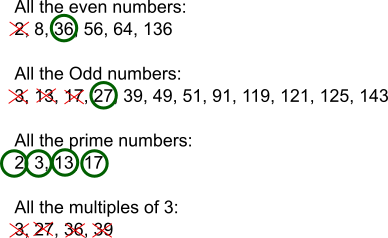#### You may also likeMake a set of numbers that use all the digits from 1 to 9, once and once only. Add them up. The result is divisible by 9. Add each of the digits in the new number. What is their sum? Now try some other possibilities for yourself!### Have You Got It?

Can you explain the strategy for winning this game with any target?### Counting Factors

Is there an efficient way to work out how many factors a large number has?

# Number Families

##### Age 11 to 14Challenge Level

Alex, Sarah and Verity from St Faith's School in the UK, Mrs Thompson's class from Broomwood Hall in the UK, Shaurya from United World College of South East Asia East Campus in Singapore, Oliver from Priory Academy LSST in the UK, Iesha from Hastings Secondary College, Westport Campus in Australia, James, Max and Jude, Himeth, Molly, Keira and Daisy and Michael, Finn and James from Priestlands School in the UK all found:
Odd numbers: $\{3, 13, 17, 27, 39, 49, 51, 91, 119, 121, 125, 143\}$
Even numbers: (or 'multiples of $2$') $\{2, 8, 36, 56, 64, 136\}$

Most of them also found
Multiples of $3$: $\{3, 27, 36, 39, 51\}$
Multiples of $4$: $\{8,36,56,64,136\}$
Multiples of $7$: $\{49,56,91,119\}$
Multiples of $8$: $\{8,56,64,136\}$

Other families that were found:
Multiples of $13$: $\{13,39,91,143\}$ (Alex, Verity, Molly, Kiera and Daisy)
Multiples of $17$: $\{17,51,119,136\}$ (Verity)
Multiples of $1$: all of the numbers (Sarah, Oliver, Iesha)
Whole numbers: all of the numbers (Mrs Thompson's class, Iesha)
Prime numbers: $\{2,3,13,17\}$ (Verity, Sarah, Mrs Thompson's class, Iesha, Himeth, Molly, Kiera and Daisy)
Composite numbers (numbers which are not prime): $\{8,27,36,39,49,51,56,64,121,125,136,143,119,121,125,136,143\}$ (Iesha, Himeth)
Square numbers: $\{36,49,64,121\}$ (Sarah, Mrs Thompson's class, Oliver, Iesha, Himeth, Molly, Kiera and Daisy)
Cube numbers: $\{8,27,64,125\}$ (Sarah, Mrs Thompson's class, Oliver, Himeth)
Triangular numbers: $\{3,36,91,136\}$ (Sarah, Oliver)
Fibonacci numbers: $\{2,3,8,13\}$ (Verity, Sarah, Himeth)

Mrs Thompson's class also found:
Numbers between $27$ and $64$: $\{39, 49, 51, 56\}$
Numbers that times by themselves equal a number less than $200$: $\{2, 3, 8, 13, 17\}$
Sum of the digits within a number equals $8$: $\{8, 17, 125, 143\}$
2-digit numbers: $\{13, 17, 27, 36, 39, 49, 51, 56, 64, 91\}$
3-digit numbers: $\{119, 121, 125, 136, 143\}$

Iesha also found:
Numbers containing $1$: $\{13,17,51,91,119,121,125,136,143\}$
Numbers starting with $1$: $\{13, 17, 119, 121, 125, 136, 143\}$
Numbers containing $2$: $\{2,27,121, 125\}$
Numbers containing $6$: $\{36,56,64,136\}$

Zoe and Ella from Priestlands School interpreted the problem so that each number could only belong to one family:
However, we could only use each number once, so we had to change some numbers to make it work. The only trouble was, we had to make sure each group had at least 4 numbers”¦

What we did:We need to use the 3 from the prime numbers because, even though it was needed for the multiple of 3 section, that section doesn't work so all the numbers can be ruled out. The same goes for 27 from odd and 36 from the even numbers.
13 and 17 couldn't be used for the odd numbers because they were needed for prime numbers.
2 from the even numbers was needed for prime numbers.
As explained before, the multiple of 3 group couldn't be used because not enough of the numbers could be used.
This solution has been changed slightly because some of Zoe and Ella's original prime numbers weren't actually prime - be careful!